Courses
Courses for Kids
Free study material
Free LIVE classes
More

# NCERT Solutions for Class 6 Maths Chapter 10 - MensurationLIVE
Join Vedantu’s FREE Mastercalss

## NCERT Solutions for Class 6 Maths Chapter 10 - Mensuration

NCERT Solutions for Class 6 Maths Chapter 10 Mensuration concentrates on providing material and also preparing students by offering different approaches for solving questions.

Since Mathematics is a highly-scoring subject, improving the performance in this subject can largely improve the overall score of students. For subjects like Science, Maths, English, and Hindi students can go through NCERT solutions.

### Important Topics Under NCERT Solutions for Class 6 Maths Chapter 10

Chapter 10 of the class 6 Maths syllabus is on Mensuration. This chapter covered in the class 6 maths syllabus has 3 major sections or topics. In order to learn from and relate to this crucial chapter in Mathematics, students are advised to go through the individual subtopics that come under this chapter. We have provided the following list of important topics that are covered under this chapter to make students get acquainted with the necessary concepts that have been used in the provided solutions for the chapter.

• Introduction

• Perimeter

1. Perimeter of Rectangle

2. Perimeter of Regular Figures

• Area

1. Area of Rectangle

2. Area of Square

We recommend that students read through each of these topics carefully and then get on to attempt and practise the NCERT Class 6 Maths Chapter 10 PDF.

Last updated date: 19th Sep 2023
Total views: 453k
Views today: 5.52k

## Access NCERT solutions for class 6 Maths Chapter 10 - Mensuration

Exercise 10.1

1. Find the perimeter of each of the following figures: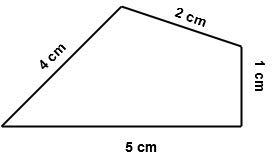Ans:  According to this figure:

As we know Perimeter= Sum of all the sides

=$1+2+4+5$

=$3+8$

=$11$

So, the perimeter= $11$ cm

2. Find the perimeter of each of the following figures: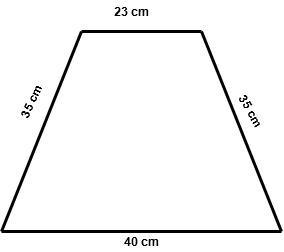Ans: According to this figure:

As we know Perimeter= Sum of all the sides

=$23+35+35+40$

=$58+75$

=$133$

So, the perimeter= $133$ cm

3. Find the perimeter of each of the following figures: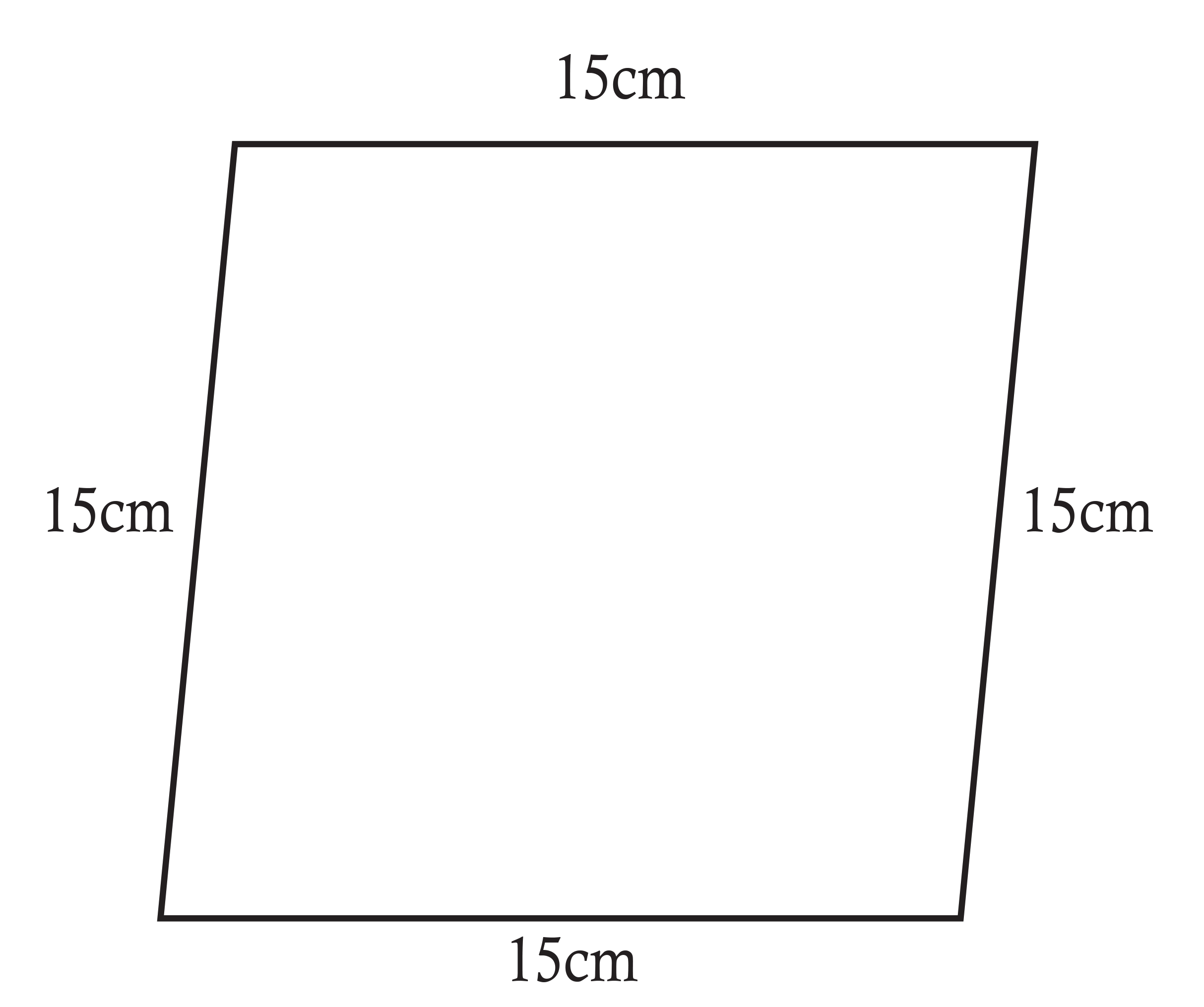Ans:  According to this figure:

As we know Perimeter= Sum of all the sides

=$15+15+15+15$

=$30+30$

=$60$

So, the perimeter= $60$ cm

4. Find the perimeter of each of the following figures: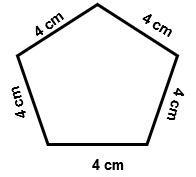Ans: According to this figure:

As we know Perimeter= Sum of all the sides

=$4+4+4+4+4$

=$8+8+4$

=$20$

So, the perimeter= $20$ cm

5. Find the perimeter of each of the following figures: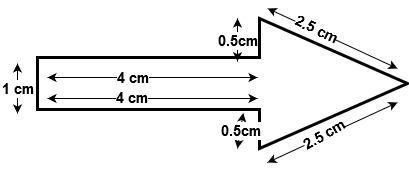Ans: According to this figure:

As we know Perimeter= Sum of all the sides

=$\text{1 + 4 + 0}\text{.5 + 2}\text{.5 + 2}\text{.5 + 0}\text{.5 + 4 }$

=$5+3+3+4$

=$8+7$

So, the perimeter= $15$ cm

6. Find the perimeter of each of the following figures: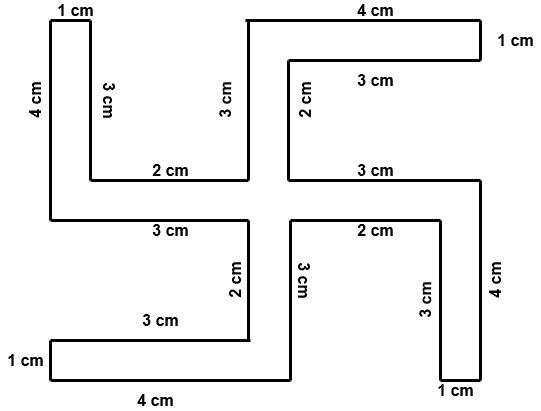Ans: According to this figure:

As we know Perimeter= Sum of all the sides

=$\text{4 + 1 + 3 + 2 + 3 + 4 + 1 + 3 + 2 + 3 + 4 + 1 + 3 + 2 + 3 + 4 + 1 + 3 + 2 + 3 }$

=$5+5+7+4+5+5+5+7+4+5$

=$10+11+10+12+9$

=$21+22+9$

=$52$

So, the perimeter= $52$ cm

7. The lid of a rectangular box of sides $40$ cm by $10$ cm is sealed all round with tape. What is the length of the tape required?

Ans: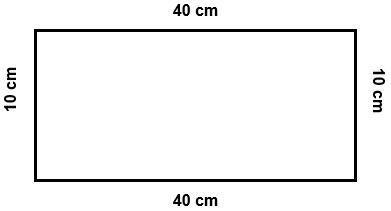Here,

Required tape length = Perimeter of rectangle

Given, Length=$40$cm

Breadth=$10$cm

=$2(length+breadth)$

= $2(40+10)$

=$2\times 50$

=$100$

Required tape length = $100$ cm.

8. A table-top measures $2$ m $25$ cm by $1$ m $50$ cm. What is the perimeter of the table-top?

Ans: Here

$25$cm = $0.25$cm

$50$cm = $0.5$cm

So, Length of table top= $2+0.25$= $2.25$m

Breadth of table top = $1+0.5$=$1.5$m

Perimeter of table top = $2(length+breadth)$

=$2(2.25+1.5)$

=$2\times 3.75$

=$7.50$m

So, Perimeter = $7.5$m

9. What is the length of the wooden strip required to frame a photograph of length and breadth $32$ cm and $21$ cm respectively?

Ans: Here

Given, Length=$32$cm

Breadth=$21$cm

Required length of wooden strip  = Perimeter of photograph

=$2(length+breadth)$

=$2(32+21)$

=$2\times 53$cm

=$106$cm

Required Length of wooden strip = $106$cm

10. A rectangular piece of land measures $0.7$ km by $0.5$ km. Each side is to be fenced with $4$ rows of wires. What is the length of the wire needed?

Ans: As we know,

Perimeter of field = $2(length+breadth)$

Given, Length=$0.7$km

Breadth=$0.5$km

=$2(length+breadth)$

=$2(0.7+0.5)$

=$2\times 1.2$km

=$2.4$km

A $4$row fence is required on each side =$4\times 2.4$= $9.6$km

So, Total length of the required field =  $9.6$km

11. Find the perimeter of each of the following shapes: A triangle of sides $3$ cm,  $4$cm and $5$ cm.

Ans: We know,

Perimeter of Triangle=$3+4+5$

=$12$cm

So, Perimeter of Triangle=$12$cm

12. Find the perimeter of each of the following shapes:  An equilateral triangle of side $9$ cm

Ans: We know,

Perimeter of Equilateral Triangle=$3\times side$

=$3\times 9$cm

= $27$cm

So, Perimeter of Equilateral  Triangle=$27$cm

13. Find the perimeter of each of the following shapes: An isosceles triangle with equal sides $8$ cm each and third side $6$ cm.

Ans: We know,

Perimeter of isosceles Triangle=$8+8+6$

=$22$cm

So, Perimeter of isosceles Triangle=$22$cm

14. Find the perimeter of a triangle with sides measuring $10$ cm, $14$ cm and $15$ cm.

Ans: We know,

Perimeter of Triangle=$10+14+15$

=$39$cm

So, Perimeter of Triangle=$39$cm

15. Find the perimeter of a regular hexagon with each side measuring $8$ cm.

Ans: We know,

Perimeter of Hexagon=$6\times 8$

=$48$m

So, Perimeter of Hexagon=$48$m

16. Find the side of the square whose perimeter is $20$ m.

Ans: We know,

Perimeter of square=$4\times side$

$20=4\times side$

Side=$\frac{20}{4}$

=$5$ m

So, Perimeter of Square=$5$m

17. The perimeter of a regular pentagon is $100$ cm. How long is its each side?

Ans: We know,

Perimeter of regular pentagon=$100$cm

$100=5\times side$

Side=$\frac{100}{5}$

=$20$ cm

So, Perimeter of Square=$20$cm

18. A piece of string is $30$ cm long. What will be the length of each side if the string is used to form: a square

Ans: We know,

Perimeter of square=$4\times side$

$30=4\times side$

Side=$\frac{30}{4}$

=$7.5$ cm

So, Perimeter of Square=$7.5$cm

19. A piece of string is $30$ cm long. What will be the length of each side if the string is used to form: an equilateral triangle

Ans: We know,

Perimeter of Equilateral Triangle=$3\times side$

$30=3\times side$

Side=$\frac{30}{3}$ cm

=$10$ cm

So, Perimeter of Equilateral Triangle=$10$cm

20. A piece of string is $30$ cm long. What will be the length of each side if the string is used to form: a regular hexagon

Ans: We know,

Perimeter of Hexagon=$30$cm

$6\times side=30$cm

Side=$\frac{30}{6}$ cm

=$5$ cm

So, Perimeter of Hexagon=$5$cm

21. Two sides of a triangle are $12$ cm and $14$ cm. The perimeter of the triangle is $36$ cm. What is the third side?

Ans: Assume third side to be $x$ cm

We know,

Perimeter of Triangle=$36$

$12+14+x=36$cm

$26+x=36$cm

$x=36-26$

$x=10$cm

So, third side =$10$cm

22. Find the cost of fencing a square park of side $250$ m at the rate of  $20$ per meter.

Ans: Given, Side of square =$250$m

We know,

Perimeter of square=$4\times side$

$=4\times 250$

=$1000$m

=$7.5$ cm

So, Perimeter of Square=$7.5$cm

Now, Cost of fencing= Rs.$20$per m

Than, for $1000$m cost of fencing = $20\times 1000$

=Rs.$20000$

23. Find the cost of fencing a rectangular park of length $175$ m and breadth $125$ m at the rate of  $12$ per meter.

Ans: Given, Length=$175$m

Breadth=$125$m

We know, Perimeter of park=$2(length+breadth)$

=$2(175+125)$

=$2\times 300$

=$600$m

Cost of fencing = $12\times 600$=Rs.$7200$

Hence, Cost of fencing = Rs.$7200$

24. Sweety runs around a square park of side $75$ m. Bulbul runs around a rectangular park with length of $60$ m and breadth $45$ m. Who covers less distance?

Ans: We know,

Perimeter of square=$4\times side$

=$4\times 75=300$m

Distance covered by Sweety =$300$m

Perimeter of rectangular park=$2(length+breadth)$

=$2(60+45)$

=$2\times 105$

=$210$m

Distance covered by Bulbul =$210$m

So, distance covered by Bulbul is less.

25. What is the perimeter of each of the following figures? What do you infer from the answer?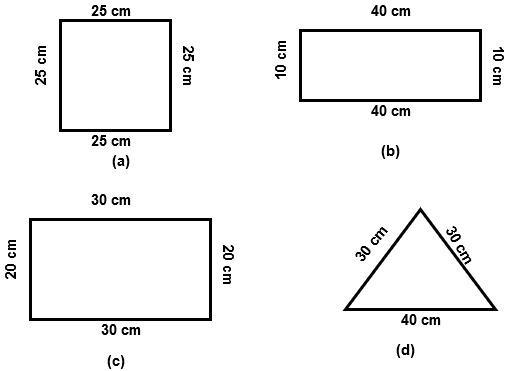Ans:

(a) We know,

Perimeter of square=$4\times side$

=$4\times 25$

=$100$cm

(b) We know,

Perimeter of rectangle=$2(length+breadth)$

=$2(40+10)$

=$2\times 50$

=$100$cm

(c) We know,

Perimeter of rectangle=$2(length+breadth)$

=$2(30+20)$

=$2\times 50$

=$100$cm

(d) We know,

Perimeter of Triangle=$30+30+40$

=$100$cm

The perimeter of each fig is the same.

26. Avneet buys $9$ square paving slabs, each with a side $\frac{1}{2}$m He lays them in the form of a square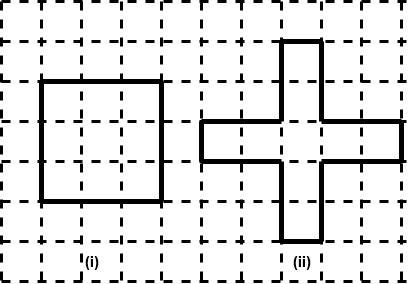(a) What is the perimeter of his arrangement?

Ans: Side of Square= $3\times side$

=$3\times \frac{1}{2}$

=$\frac{3}{2}$m

Perimeter of Square = $4\times \frac{3}{2}$

=$2\times 3$

=$6$m

(b) Shari does not like his arrangement. She gets him to lay them out like a cross. What is the perimeter of her arrangement?

Ans: Perimeter = $\text{ 0}\text{.5 + 1 + 1 + 0}\text{.5 + 1 + 1+ 0}\text{.5 + 1 + 1 + 0}\text{.5 + 1 + }1$

=$10$m

(c) Which has greater perimeter?

Ans: Cross arrangement has greater perimeter.

(d) Avneet wonders, if there is a way of getting an even greater perimeter. Can you find a way of doing this? (The paving slabs must meet along complete edges, i.e., they cannot be broken.)

Ans: Yes, the perimeter will be 10 cm if all of the squares are lined up in a straight line.

Exercise 10.2

1. Find the areas of the following figures by counting squares: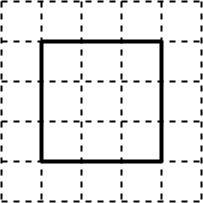Ans:  Here,

The number of squares is $9$

So, Area = $9$ sq. units

2. Find the areas of the following figures by counting squares: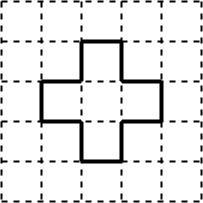Ans:  Here,

The number of squares is $5$

So, Area = $5$ sq. units

3. Find the areas of the following figures by counting squares: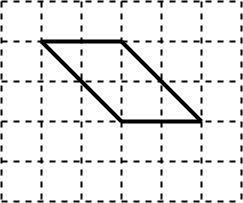Ans:  Here,

The number of complete squares is $2$

The number of half squares is $4$

So,  Total Area = $2+4(0.5)=4$ sq. units

4. Find the areas of the following figures by counting squares: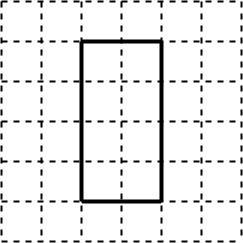Ans: Here,

The number of squares is $8$

So, Area = $8$ sq. units

5. Find the areas of the following figures by counting squares: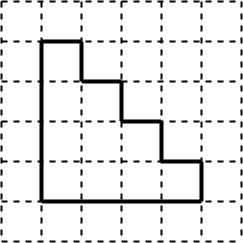Ans: Here,

The number of squares is $10$

So, Area = $10$ sq. units

6. Find the areas of the following figures by counting squares: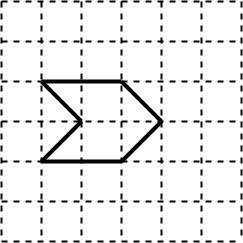Ans: Here,

The number of complete squares is $2$

The number of half squares is $4$

So,  Total Area = $2+4(0.5)=4$ sq. units

7. Find the areas of the following figures by counting squares: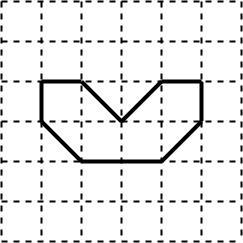Ans: Here,

The number of complete squares is $4$

The number of half squares is $4$

So,  Total Area = $4+4(0.5)=6$ sq. units

8. Find the areas of the following figures by counting squares: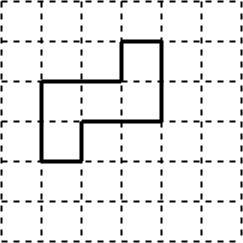Ans: Here,

The number of squares is $5$

So, Area = $5$ sq. units

9. Find the areas of the following figures by counting squares: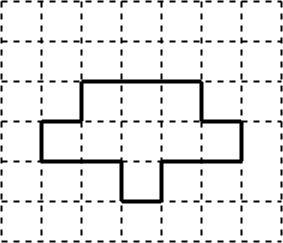Ans: Here,

The number of squares is $9$

So, Area = $9$ sq. units

10. Find the areas of the following figures by counting squares: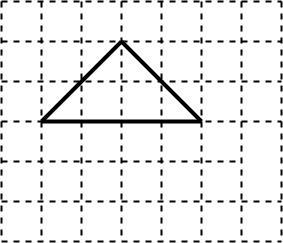Ans: Here,

The number of complete squares is $2$

The number of half squares is $4$

So,  Total Area = $2+4(0.5)=4$ sq. units

11. Find the areas of the following figures by counting squares: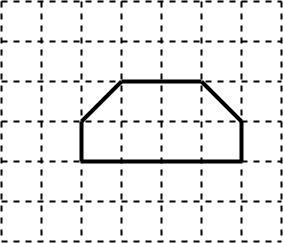Ans: Here,

The number of complete squares is $4$

The number of half squares is $2$

So,  Total Area = $4+2(0.5)=5$ sq. units

12. Find the areas of the following figures by counting squares: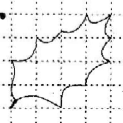Ans: Here,

The number of complete squares is $3$

The number of half squares is $10$

So,  Total Area = $3+10(0.5)=8$ sq. units

13. Find the areas of the following figures by counting squares: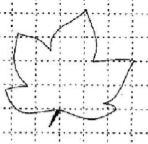Ans: Here,

The number of complete squares is $7$

The number of half squares is $14$

So, Total Area = $7+14(0.5)=14$ sq. units

14. Find the areas of the following figures by counting squares: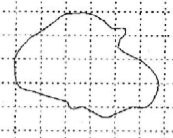Ans:  Here,

The number of complete squares is $10$

The number of half squares is $16$

So,  Total Area = $10+16(0.5)=18$ sq. units

Exercise 10.3

1. Find the areas of the rectangles whose sides are:$3$cm and $4$cm

Ans: We know, the area of triangle = Length $\times$ Breadth

Here,

Length=$3$cm

Breadth=$4$cm

Area=$3\times 4$= $12$cm2

2. Find the areas of the rectangles whose sides are:$12$m and $21$m

Ans: We know, the area of triangle = Length $\times$ Breadth

Here,

Length=$12$m

Breadth=$21$m

Area=$12\times 21$= $252$m2

3. Find the areas of the rectangles whose sides are:$2$km and $3$km

Ans: We know, the area of triangle = Length $\times$ Breadth

Here,

Length=$2$km

Breadth=$3$km

Area=$2\times 3$= $6$km2

4. Find the areas of the rectangles whose sides are:$2$m and $70$cm

Ans: We know, the area of triangle = Length $\times$ Breadth

Here,

Length=$2$m

Breadth=$70$cm = $0.7$m

Area=$2\times 0.7$= $1.4$m2

5. Find the areas of the squares whose sides are: $10$cm

Ans: We know,

Area of square = Side2

=${{10}^{2}}$

=$100$ cm2

6. Find the areas of the squares whose sides are: $14$cm

Ans: We know,

Area of square = Side2

=${{14}^{2}}$

=$196$ cm2

7. Find the areas of the squares whose sides are: $5$cm

Ans: We know,

Area of square = Side2

=${{5}^{2}}$

=$25$ m2

8. The length and the breadth of three rectangles are as given below:

(a) $9$m and $6$m  (b) $17$m and $3$m  (c) $4$m and $14$m

Which one has the largest area and which one has the smallest?

Ans:

(a) We know, the area of rectangle = Length $\times$ Breadth

Here,

Length=$9$m

Breadth=$6$m

Area=$9\times 6$= $54$m2

(b) We know, the area of rectangle = Length $\times$ Breadth

Here,

Length=$3$m

Breadth=$17$m

Area=$3\times 17$= $51$m2

(c) We know, the area of rectangle = Length $\times$ Breadth

Here,

Length=$4$m

Breadth=$14$m

Area=$4\times 14$= $56$m2

Rectangle (c) has the largest size, $56$ m2, whereas rectangle (b) has the smallest area, $51$ m2

9. The area of a rectangle garden $50$ m long is $300$ m2, find the width of the garden.

Ans: We know, the area of rectangle = Length $\times$ Breadth

Here,

Area of rectangle =$300$ m2

Length= $50$m

$300=50\times width$

Width=$6$m

10. What is the cost of tilling a rectangular plot of land $500$ m long and $200$ m wide at the rate of ` $8$ per hundred sq. m?

Ans: Area of the land can be calculated as

=$500\times 200$

=$1,00,000$m2

Cost of tiling $1,00,000$m2 of land will be

=$\frac{8\times 100000}{100}$ = Rs.$8000$

11. A table-top measures $2$ m by $1$ m $50$ cm. What is its area in square meters?

Ans: Given,

L=$2$m

B=$1$m$50$cm= $1.5$m

Area = L$\times$B

=$2\times 1.50=3$m2

12. A room us $4$ m long and $3$ m $50$ cm wide. How many square meters of carpet is needed to cover the floor of the room?

Ans:: Given,

L=$4$m

B=$3$m$50$cm= $3.5$m

Area = L$\times$B

=$4\times 3.50=14$m2

13. A floor is $5$ m long and $4$ m wide. A square carpet of sides $3$ m is laid on the floor. Find the area of the floor that is not carpeted.

Ans:  Here,

Area of floor = L$\times$B=$5$ m $\times$ $4$ m = $20$ m2

Area of square carpet = $3$ $\times$ $3$ = $9$ m2

Area of floor that is not carpeted =  $20-9$=$11$ m2

14. Five square flower beds each of sides $1$ m are dug on a piece of land $5$ m long and $4$ m wide. What is the area of the remaining part of the land?

Ans: Here,

Area of flower square bed =$1\times 1=1$ m2

Area of 5 square beds = $1\times 5=5$ m2

Area of land = $5\times 4=20$m2

Remaining Area = Area of land – Area of 5 flower beds = $20-5=15$ m2

Therefore, remaining part of the land= $15$m2

15. By splitting the following figures into rectangles, find their areas. (The measures are given in centimeters)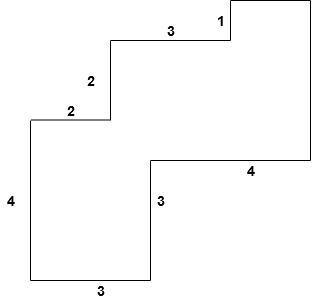Ans:

Here,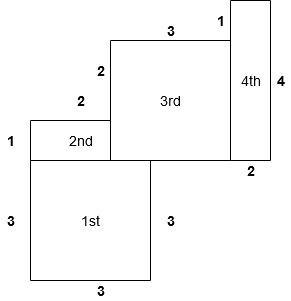Area of 1st region =$3\times 3=9$ cm2

Area of 2nd region = $1\times 2=2$cm2

Area of 3rd region = $3\times 3=9$ cm2

Area of 4th region = $2\times 4=8$ cm2

Hence, Total area = $9+2+9+8$ = $28$ cm2

16. By splitting the following figures into rectangles, find their areas. (The measures are given in centimeters)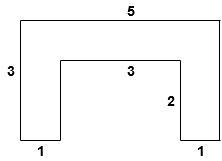Ans: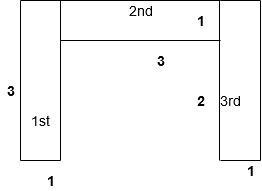Area of 1st region =$3\times 1=3$ cm2

Area of 2nd region = $3\times 1=3$cm2

Area of 3rd region = $3\times 1=3$ cm2

Hence, Total area = $3+3+3$ = $9$ cm2

17. Split the following shapes into rectangles and find their areas. (The measures are given in centimeters)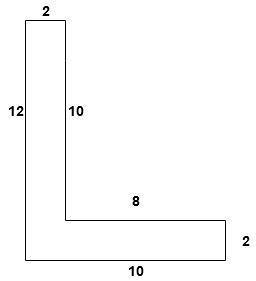Ans: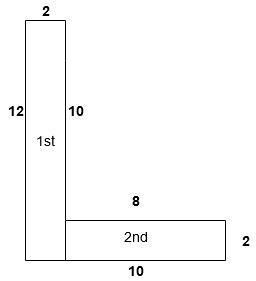Here,

Area of 1st rectangle= $12\times 2$

Area of 2nd rectangle= $8\times 2$

Total area of the figure = $12\times 2+8\times 2$ = $40$ cm2

18. Split the following shapes into rectangles and find their areas. (The measures are given in centimeters)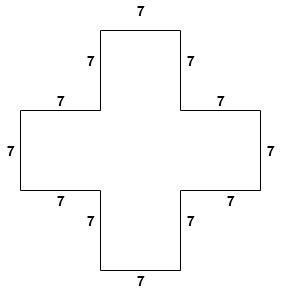Ans: Here,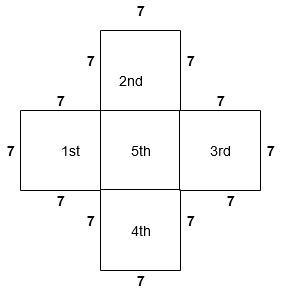From figure it is clear that there are five square with each side  $7$.

Area of one square=$7\times 7=49$

So, area of five square= $49\times 5=245$cm2

19. Split the following shapes into rectangles and find their areas. (The measures are given in centimeters)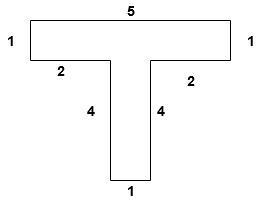Ans: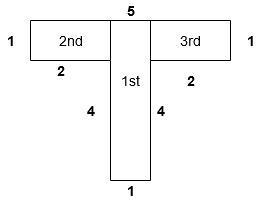Area of 1st region = $5\times 1=5$cm2

Area of 2nd region =$2\times 1=2$ cm2

Area of 3rd region = $2\times 1=2$ cm2

Hence, Total area = $2+5+2$ = $9$ cm2

20. How many tiles whose length and breadth are $12$ cm and $5$ cm respectively will be needed to fit in a rectangular region whose length and breadth are respectively:$100$ cm and $144$ cm

Ans: Here,

Area of Rectangle= $100\times 144=14400$cm2

Area of one tile = $5\times 12=60$cm2

We know, Number of tiles = Area of rectangle / Area of one tile

=$\frac{14400}{60}$

= $240$cm2

$240$ tiles are required.

21. How many tiles whose length and breadth are $12$ cm and $5$ cm respectively will be needed to fit in a rectangular region whose length and breadth are respectively:$70$ cm and $36$ cm

Ans: Here,

Area of Rectangle= $70\times 36=2520$cm2

Area of one tile = $5\times 12=60$cm2

We know, Number of tiles = Area of rectangle / Area of one tile

=$\frac{2520}{60}$

= $42$cm2

$42$ tiles are required.

• Curated by Vedantu’s Maths wizards, solutions of NCERT Class 6 Maths Chapter 10 PDF guides the students towards the best problem-solving approaches.

• Attributing to the different approaches used, students can develop a better perspective on the overall Mensuration chapter.

• The Class 6 Maths Chapter 10 worksheet PDF aids students in understanding the fundamentals of the topics so that the understanding of important concepts is strengthened.

## NCERT Solutions for Class 6 Maths Chapter 10 - Mensuration

### 10.1 Introduction

The solutions of NCERT for Class 6 Chapter 10 Mensuration PDF aims to recall the concept of plain shapes which the students have learned in the third grade. In this chapter, students will learn about the boundaries, and perimeters of the plane figures. It broadens to cover the comparison of various shapes in terms of measurements.

### 10.2 Perimeter

• Chapter 10 Class 6 of Maths introduces the concept of the perimeter to the students. It offers a detailed explanation by showing figures and comparing them with the wire or string.

• According to the NCERT Solutions of Class 6th Chapter 10 Maths, the perimeter can be defined as the distance covered along the boundary forming a closed figure.

• Also, the Class 6 Chapter 10 worksheet PDF has four unsolved questions to assess the students and to know how far they understood the concept.

10.2.2 Perimeter of a Rectangle

Class 6 Mensuration extendes the topic of the perimeter to to include the rectangle. As the students must be aware that the opposite sides of a rectangle are equal, they can easily find the perimeter by taking the measurements of both the sides in which one is called the length and the other is called the breadth. The formula to be used is:

For a better understanding of students, the NCERT solutions of Maths Class 6 provides five solved examples.

### 10.2.3 Perimeter of Regular figures

• NCERT Maths book Class 6 Chapter 10 Mensuration allows the students to learn more about the concept of perimeter. In this section, the students are asked to consider the regular shapes which they may come across in their surroundings.

• It can be a square, rectangle, or triangle, etc. There will be a separate formula to find out the perimeter of each particular figure. For instance, if you want to find out the perimeter of a square, it has four equal sides. Then the perimeter of the square will be,

Perimeter = 4 * length of the side.

• Similarly, if the students want to find the perimeter for a triangle such as an equilateral triangle that has all three sides equal, then the perimeter can be calculated by using the below formula:

Perimeter = 3 * length of side.

### 10.3 Area

• The NCERT Solutions of Maths Class 6 Chapter 10 Mensuration covers the concept of area as well.

• Students get to learn about what is the area of the shape and how it can be calculated for various shapes.

• Area can be defined as the amount of surface enclosed by a closed figure.

• NCERT lists a few sums for students to try the good practical knowledge on finding the area of figures.

10.3.1 Area of Rectangle

In this section, students can learn how to find the area of a rectangle by considering the values of length and breadth. Similar to the perimeter, the area of the rectangle also has a formula which is given below:

Area of Rectangle = length * breadth.

10.3.2 Area of Square

• Students can calculate the area of a square using graph paper or plain paper.

• The NCERT Class 6 Mensuration solutions offers a set of five solved examples to understand this concept.

• As the students already learned about the perimeter of a square, it is easy to find the area of a square of side ‘s’ using the below formula.

Area of a square =length of the side (s) * length of the side (s).

### NCERT Solution Class 6 Maths of Chapter 10 All Exercises

Chapter 10 - Mensuration Exercises in PDF Format

Exercise 10.1

17 Questions with Solutions

Exercise 10.2

1 Questions with Solutions

Exercise 10.3

12 Questions with Solutions

## Key Features of NCERT Solutions Class - 6 Maths Chapter - 10

• Solutions of NCERT Class 6 Maths Chapter 10 PDF helps to enrich their knowledge and to improve their scores.

• Our NCERT Solutions provide a free download for PDF as well as several subject experts are ready to clarify the doubts of students through live chat are in a chatbox on the official website.

• It is useful to gain a sound knowledge as well as to participate in the Olympiads and national level talent test etc.

• Free download PDF helps the students to revise and recollect the information whenever they want.

## Preparation Tips For Class 6 Maths Mensuration Chapter

To help students get a better understanding of the Mensuration concepts, we provide the NCERT Solutions for Class 6 Mensuration Chapter PDF free of cost. Inculcate the following tips to score higher-

• Understand the fundamentals of Mensuration by going through the definitions.

• Prepare a separate notebook to jot down all the formulas of Mensuration. Go through it regularly to memorize it.

• Solve the NCERT Class 6 Mensuration questions on a regular basis. Take the help of the solutions PDF to learn various approaches.

• Include the questions of all sub-topics related to chapter so as to not miss out on any topic.

• Take the aid of revision notes to refresh the concepts and retain the formulas.

### Chapter Based NCERT Solutions for Class 6 Maths

Along with this, students can also view additional study materials, for Class 6 Maths Chapter 1 Knowing Our Numbers

With the Class 6 Chapter 10 NCERT Solutions, get rid of all your doubts and bolster your understanding of the fundamental concepts. Delve deeper into the detailed explanations and develop a perspective for varying approaches that can be used towards solving Mensuration related questions. Practice the exercises thoroughly to understand the intricricaties. You can visit our website for more NCERT solutions.

## FAQs on NCERT Solutions for Class 6 Maths Chapter 10 - Mensuration

1. Calculate the Perimeter of a Square Which is 3 cm as its Length of the Side.

The length of a side = 3 cms

The formula for calculating perimeter of a square = 4* side

= 4*3

= 12 cms

Therefore, Perimeter = 12 cms.

2. Is it easy to Learn Mathematics Using These PDF solutions?

Yes. It is easy to learn mathematics using NCERT PDF books even for the slow learners because every concept has been explained in a detailed manner by a group of well-experienced experts. They provide several solid examples along with the unsolved test papers. It makes the students get a sound knowledge of every concept. If the students have any doubts, the teachers are available on the website to clarify the doubts.

3. What is the tangent of a circle?

A tangent to a circle is a straight line that meets the circle at anyone or only one point, such point is known as tangency, and at this point, the tangent is at 90 degrees with respect to the circle’s radius. Tangent lines to circles are the topic of numerous theorems and are used in a variety of geometrical constructions and proofs.

4. Is NCERT Class 6 Maths chapter 10 easy?

Maths is fun if you know the tricks, but if you don't know the topics and formulae well, things can get complicated and confusing because there are numerous ways to solve a question or equation. This chapter on Mensuration, Chapter 10 Class 6, is a very important one, and it will also form the base for the other chapters that you will learn further. Focus on the fundamentals, and with enough practise, you'll be fine.

5. What do you mean by a minor and a major arc?

A minor arc is the shortest arc that connects two points on a circle. A minor arc has a measure that is less than 180° and equal to the measure of the arc's centre angle. The longer arc connecting two endpoints of a circle is known as a major arc. These two are fundamental parts of a circle and must be learnt well to solve different problems related to circles.

6. How to achieve good marks in Class 6 Maths Chapter 10?

The steps one can take would be to ace well in Class 6 Chapter 10:

• Refresh your memory on the essentials. Try to recall all the basic concepts.

• Go for the basic logic behind any derivation and formula. Learn each formula related to circles judiciously.

• Comprehensively understand the different approaches to solving any equation or any problem.

• Try to answer NCERT questions.

• Determine which topics of Mensuration you are acquainted with and which require additional practice.

• If you are experiencing difficulties comprehending a topic, you may seek the help of NCERT Solutions for Class 6 Maths to remove any doubts.

7. What do you mean by Mensuration?

Mensuration is a branch of mathematics that examines the computation of geometric figures and their characteristics such as area, length, volume, lateral surface area, surface area, and so on. It explains all of the basic equations and characteristics of numerous geometric forms and figures, as well as the foundations of computation. It is an important aspect of Mathematics, and proper attention must be given to this topic along with adequate practice.